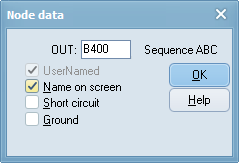﻿ The graphical user interface > Circuit Objects > Customizing Components > Open Node dialog

# Open Node dialog

Navigation:  The graphical user interface > Circuit Objects > Customizing Components >

# Open Node dialog

In the Open Node dialog you specify data for a single component node. There are four different kinds of nodes; each treated slightly different in this dialog box.

Parameters common to all nodes are:

The phase sequence appears after compile circuit is performed (ATP|Run ATP or ATP|Sub-process/Make node names). Transposition is only possible for 3-phase nodes. A four-phase node will have the phase sequence A..D. The user can connect a single phase connection to this node and specify which phase to connect to in the Connection dialog.

The following list explains the type specific node parameters.

Standard and user specified component node:

Ground If checked, the node is grounded. Click on the ground symbol to rotate it.The Open Node dialog box for standard and user specified components.

Model node:

TACS node:Type 0=Output.

1=Input signal positive sum up.

2=Input signal negative sum up.

3=Input signal disconnected.

(only necessary if node name is user specified)

TACS controlled machine node:

Type 0=No control.

1=D-axis armature current. Out.

2=Q-axis armature current. Out.

3=Zero-sequence armature current. Out.

4=Field winding current. Out.

5=D-axis damper current. Out.

6=Current in eddy-current winding. Out.

7=Q-axis damper current. Out.

8=Voltage applied to d-axis. Out.

9=Voltage applied to q-axis. Out.

10=Zero-sequence voltage. Out.

11=Voltage applied to field winding. Out.

12=Total mmf in the machines air-gap. Out.

13=Angle between q- and d-axis component of mmf. Out.

14=Electromagnetic torque of the machine. Out.

15=Not in use.